# Decimal Place Value Lesson Plan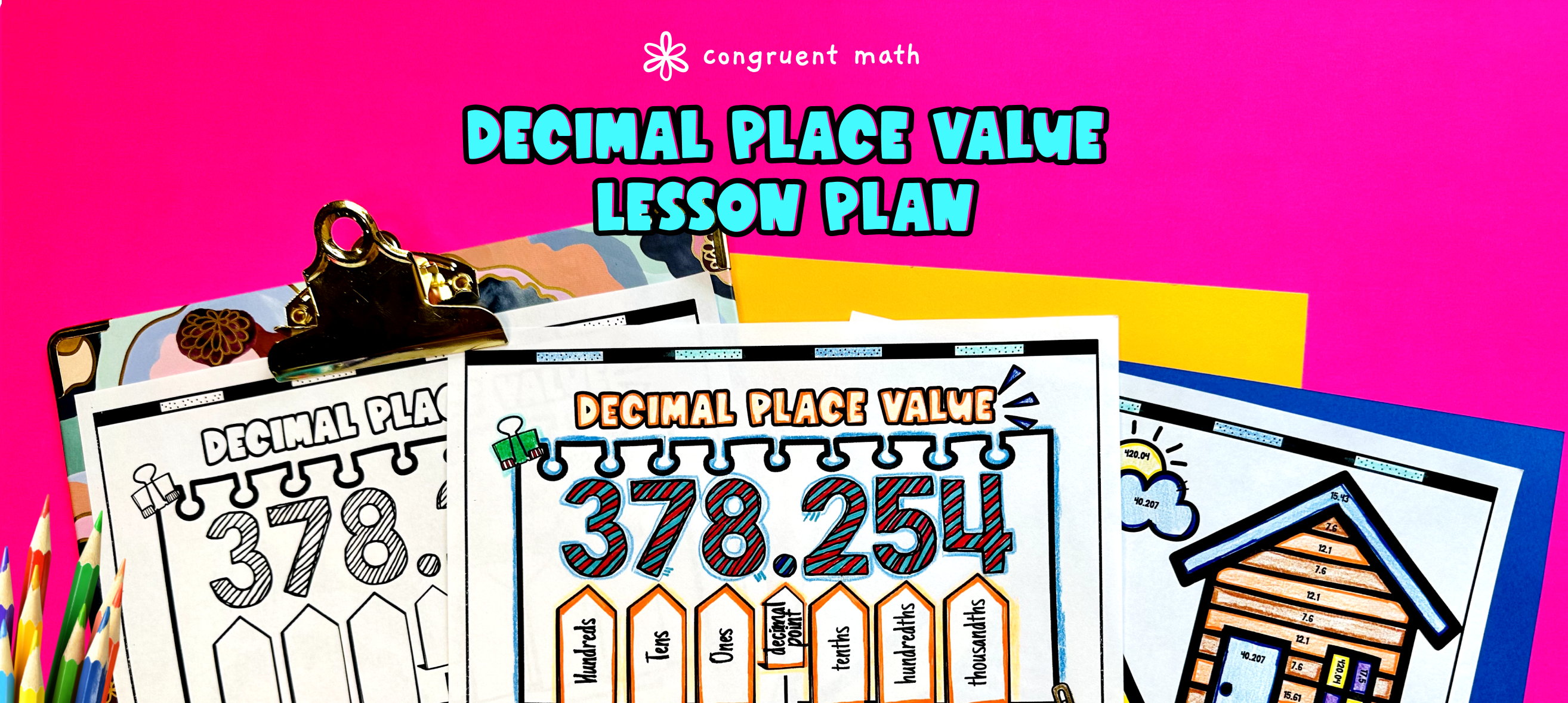Pin This

## Overview

Ever wondered how to teach decimal place value in an engaging way to your 5th and 6th grade students?

In this lesson plan, students will learn about the value of decimals and their real-life applications. Through artistic, interactive guided notes, check for understanding, a color by code activity, and a real-life application example, students will gain a comprehensive understanding of decimal place value.

The lesson culminates with an exploration of online shopping with video games, highlighting the importance of understanding decimal place value in real-world scenarios.

## Get the Lesson Materials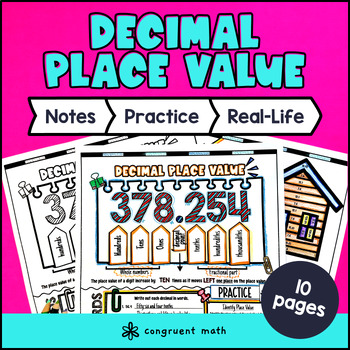\$4.25

## Learning Objectives

After this lesson, students will be able to:

• Identify the place value of decimals up to thousandths

• Convert between standard and expanded form of decimals

• Round decimals to the nearest whole number, tenth, and hundredth

• Order decimals from least to greatest and greatest to least

• Compare decimals using the greater than, less than, and equal to symbols

• Understand the real-life applications of decimal place value in online shopping with video games

## Prerequisites

Before this lesson, students should be familiar with:

• Basic math operations (addition, subtraction, multiplication, and division)

• Basic understanding of fractions and decimals (optional, but helpful)

• Understanding of place value in whole numbers (up to thousands)

## Key Vocabulary

• Decimal place value chart

• Standard form

• Expanded form

• Rounding

• Ordering

• Comparing

• Tenths

• Hundredths

• Thousandths

## Procedure

### Introduction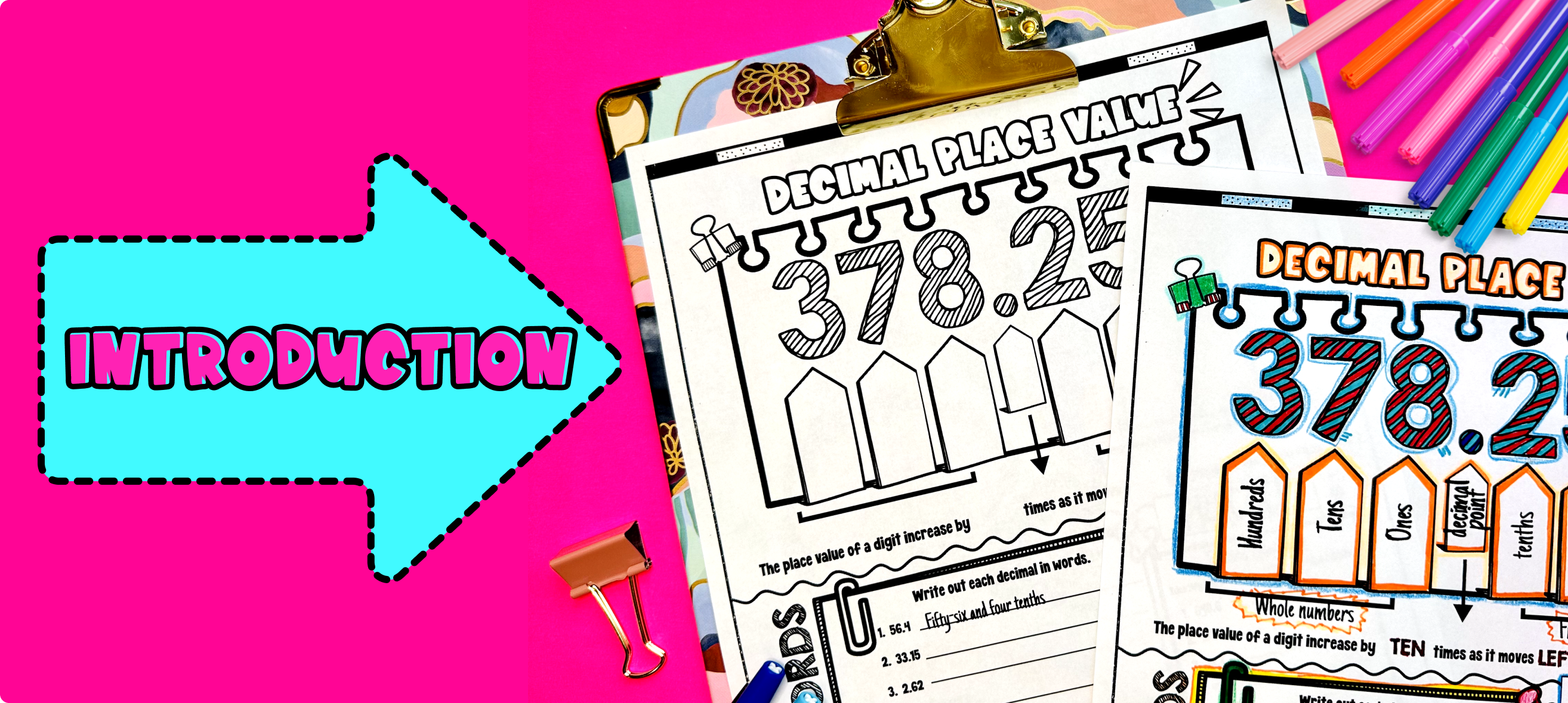Pin This

As a hook, ask students why understanding decimal place value is important in real-life scenarios. Throughout this lesson plan, students will learn about the value of decimals and their real-life applications. They will gain a comprehensive understanding of decimal place value through artistic, interactive guided notes, check for understanding, a color by code activity, and a real-life application example.

Use the guided notes to introduce the following topics:

• Identifying place up to thousandths

• Converting between standard and expanded form

• Rounding decimals

• Ordering decimals

• Comparing decimals

Walk through the key points of each topic to teach. Refer to the FAQ below for a walk through on this, as well as ideas on how to respond to common student questions.

Along the way as checks for understanding, have students walk through the “You Try!” sections. Call on students to talk through their answers, potentially on the whiteboard or projector. Based on student responses, reteach concepts that students need extra help with.

### Practice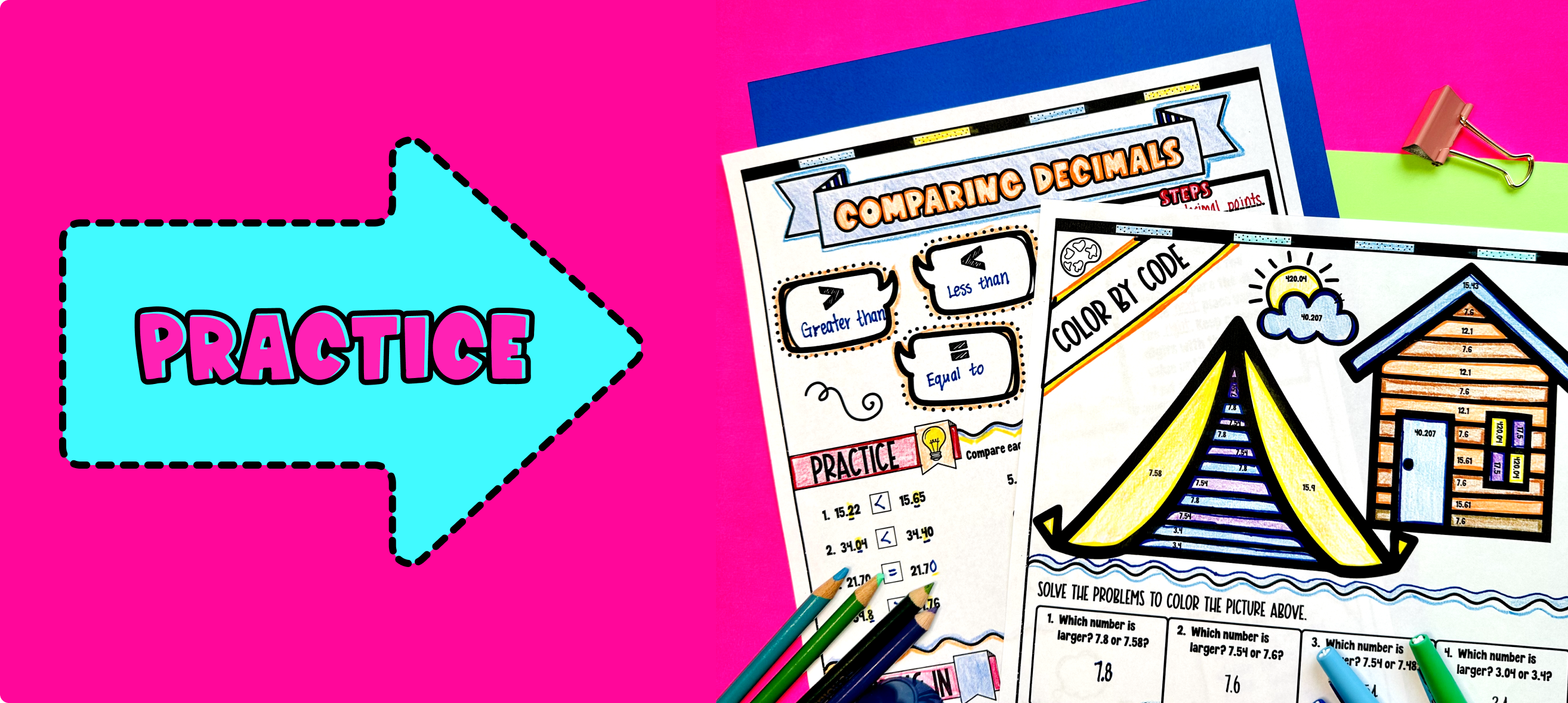Pin This

Have students practice identifying and ordering decimals using the decimal place value color by code activity. This activity engages students with a fun and interactive way to practice the concepts learned in the guided notes.

Walk around to answer student questions and provide support as needed.

### Real-Life Application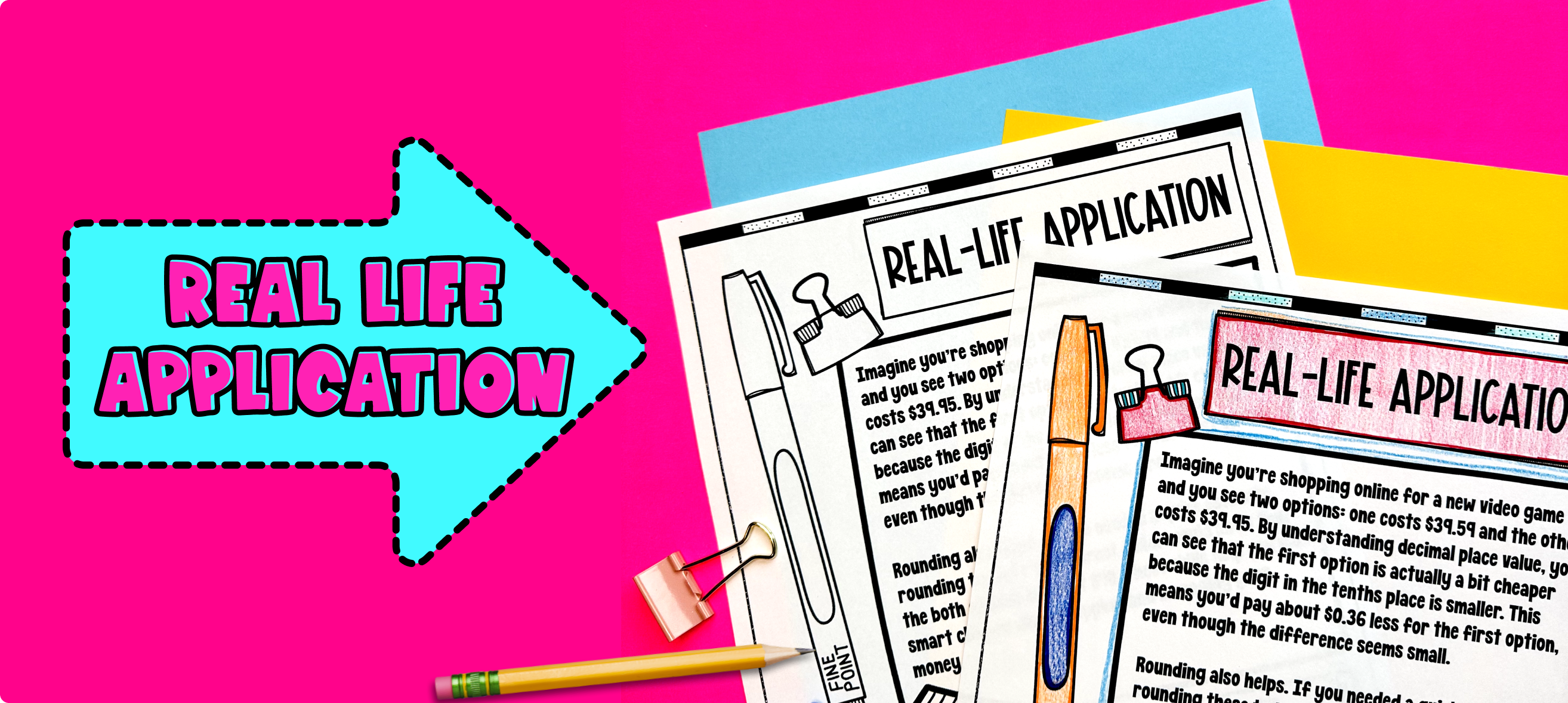Pin This

Bring the class back together, and introduce the concept of online shopping with video games. Discuss how video games often have in-game purchases and the importance of understanding decimal place value when making purchases. Ask students to brainstorm different scenarios in which understanding decimal place value is important when shopping online for video games.

Refer to the FAQ below for more ideas on how to teach the real-life application of decimal place value in online shopping with video games.